Search IntMath
Close

450+ Math Lessons written by Math Professors and Teachers

5 Million+ Students Helped Each Year

1200+ Articles Written by Math Educators and Enthusiasts

Simplifying and Teaching Math for Over 23 Years

# The IntMath Newsletter - 20th July 2008

By Murray Bourne, 20 Jul 2008

1. Current math events
2. Math tips - (a) Math Dictionary; (b) Numerical methods
3. Poll results - Summer math
4. From the math blog

## 1. Current math events

I have been running a series of articles in the squareCircleZ blog on recent applications of math:

For a really extensive list of recent research and developments in math (56,000 articles), check out ScienceDaily.

## 2. Math Tips

(a) Looking for a Math Dictionary?
Each new topic in math involves many new vocabulary terms. Do you have a brain like a sieve when it comes to math definitions? This free Math Dictionary from MathWords.com may be just the thing. There is an alphabetical list of math words as well as definitions grouped by topic, including numbers & symbols, geometry, algebra, trigonometry, calculus, probability & statistics, and real world applications.

I hope you find it useful.

(b) Numerical Methods
Have you ever wondered how the early mathematicians developed the formulas that we use every day?

Usually there was some important problem that needed solving and the best the mathematicians could do was to approximate the answer using numerical methods.

For example, how would you find the area of a circle if you didn't know the formula A = πr2?

As far back as the 3rd century BCE (over 2,200 years ago), the Greek mathematician Archimedes worked out a remarkably accurate value for the area of a circle. He was aiming to find a square that had the same area as a given circle. This process is known as "squaring the circle".

Archimedes approached the problem by drawing a square inside a circle, so that the 4 corners of the square touch the circle. The area of that square is easy to find (actually, the square roots involved gave him some trouble), but its area is clearly smaller than the area of the circle as you can see in this image.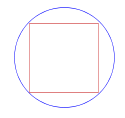He then figured that if you draw another square outside the circle, so the circle just touches the 4 sides of the square, then the area of the circle must be somewhere between the areas of the smaller and larger squares.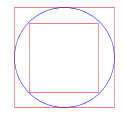He had a reasonable approximation for the area of the circle by taking the average of the areas of smaller and larger squares.

To improve on this, the next step was to draw a regular pentagon inside the circle. As we can see in the image, the area of the pentagon is closer to the area of the circle, but still too small.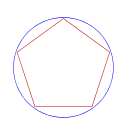Once again, we draw a larger pentagon outside the circle and find its area.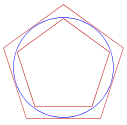Our desired area is somewhere in between the areas of the 2 pentagons.

We can keep going with this and each time we do it, we get a better approximation for the area of our circle. Here's what a dodecahedron (12 sided polygon) looks like inside the circle.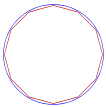You can see that the area of the dodecahedron is getting quite close to the area of the circle. What Archimedes has done is to use a numerical approach to find the area of the circle.

You can see more on this topic, with calculations included here.

[BTW, the topic of "squaring the circle" is where this math blog gets its name: "squareCircleZ".]

## 3. Poll results - summer math

The most recent IntMath poll asked readers "How much school-related study will you do during summer?". Just over 40% indicated that they were going to summer school, which is higher than I expected, but probably not that surprising since if you are doing math at summer school, you'll need to visit Interactive Mathematics. The rest indicated between zero study and "maybe a week".

The results for "how much study will you do in summer?":

43% I'm going to summer school
20% Maybe 1 week
20% None
11% Maybe 1 hour
7% Maybe 1 day

The current poll asks you about how inflation is affecting you personally. You can vote on any page in Interactive Mathematics.

## 4. From the math blog

1) Friday Math Movie - I Will Derive
This week's movie is a fun summary of when and how to apply differentiation.

2) EduSim - free 3D multi-user virtual world
EduSim is a 3-D virtual world that comes as a free download. It's worth checking it out.

3) Google search, math and latent semantic analysis
There is sophisticated math going on behind the scenes when you perform a search on Google. It involves a technique called latent semantic analysis which is based on matrix operations.

4) Friday Math Movie - The Amazing Origami of Robert Lang
An origami specialist talks about how math helps him to create beautiful art.

5) Math in computer game development
Behind every computer game lie many applications of mathematics.

## 5. Your brain and math

Last newsletter, I linked to a special article on Math problem solving and brain activity.

Thanks to all the readers who wrote giving feedback that they found the article very interesting and useful.

It is quite important that teachers — and students — understand how the brain deals with math and where the stumbling blocks can occur. In this age where there are thousands of Weapons of Math Distraction and we all suffer from attention deficit disorder, brain research helps us to understand how we process problems and what we give our attention to, and why.

If you missed the article last time, here's the link again: Math problem solving and brain activity.

Till next time.

Be the first to comment below.

### Comment Preview

HTML: You can use simple tags like <b>, <a href="...">, etc.

To enter math, you can can either:

1. Use simple calculator-like input in the following format (surround your math in backticks, or qq on tablet or phone):
a^2 = sqrt(b^2 + c^2)
(See more on ASCIIMath syntax); or
2. Use simple LaTeX in the following format. Surround your math with $$ and $$.
$$\int g dx = \sqrt{\frac{a}{b}}$$
(This is standard simple LaTeX.)

NOTE: You can mix both types of math entry in your comment.